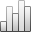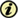Acta Stereologica

0351-580XMore Statisticssince 05 December 2013 :
View(s): 375 (1 ULiège)
Lars M. Karlsson & Arun M. Gokhale

### Estimating mean linear intercept length using the trisector

(Volume 15 (1996) — Number 1 - Mathematical foundations of stereology - Feb. 1996)
Article#### Abstract

We propose a method for estimating the mean linear intercept length L, of anisotropic, space-filling grains or cells. The practical sampling and estimation procedure is summarized in the following steps. (1) Take a vertical axis perpendicular to the axis of structural anisotropy. (2) Take three vertical sections mutually at an angle of 120° apart, and parallel to the vertical axis. The first vertical section is randomly oriented around the vertical axis. This probe is called a trisector. (3) Overlay a. test system of cycloid arcs and points on each of the three vertical sections, and align the cycloid minor axes parallel to the vertical direction. The vertical direction must be identifiable on the vertical sections. (4) Count the total number of intersections between the cycloids and the traces of the surfaces of interest, and the total number of test points that hit the reference space. (5) Calculate an estimate of L as the ratio of total point to total intersection counts, times a constant characteristic of the test system used. We estimated the mean linear grain size of a rolled plate of an extra-low-carbon steel to 20µm, and assessed an upper limit for the coefficient of error of this mean to about 2%. Our conclusion is, that the trisector is an unbiased and precise method for estimating the mean linear intercept length of space-filling, anisotropic grains or cells. With a small modification of the estimator of L, the proposed method may also be applied to anisotropic, separated objects.

Keywords : stereology, anisotropy, cycloid, ELC-steel. vertical sections, trisector